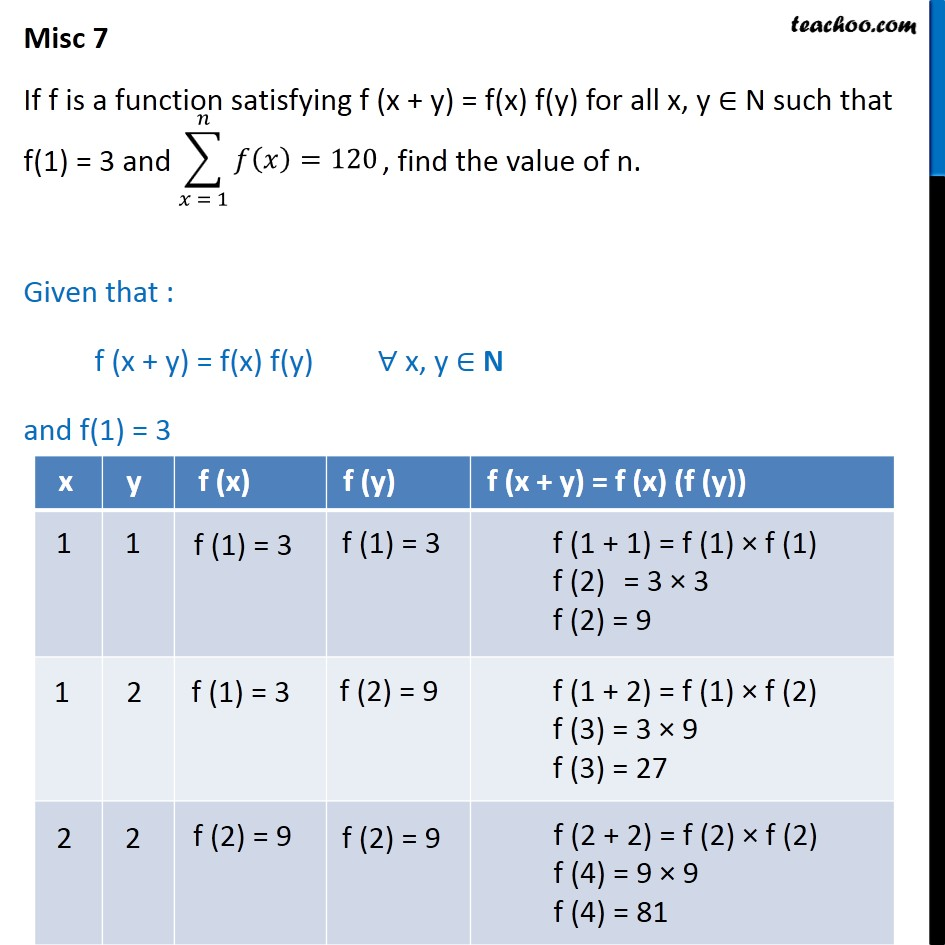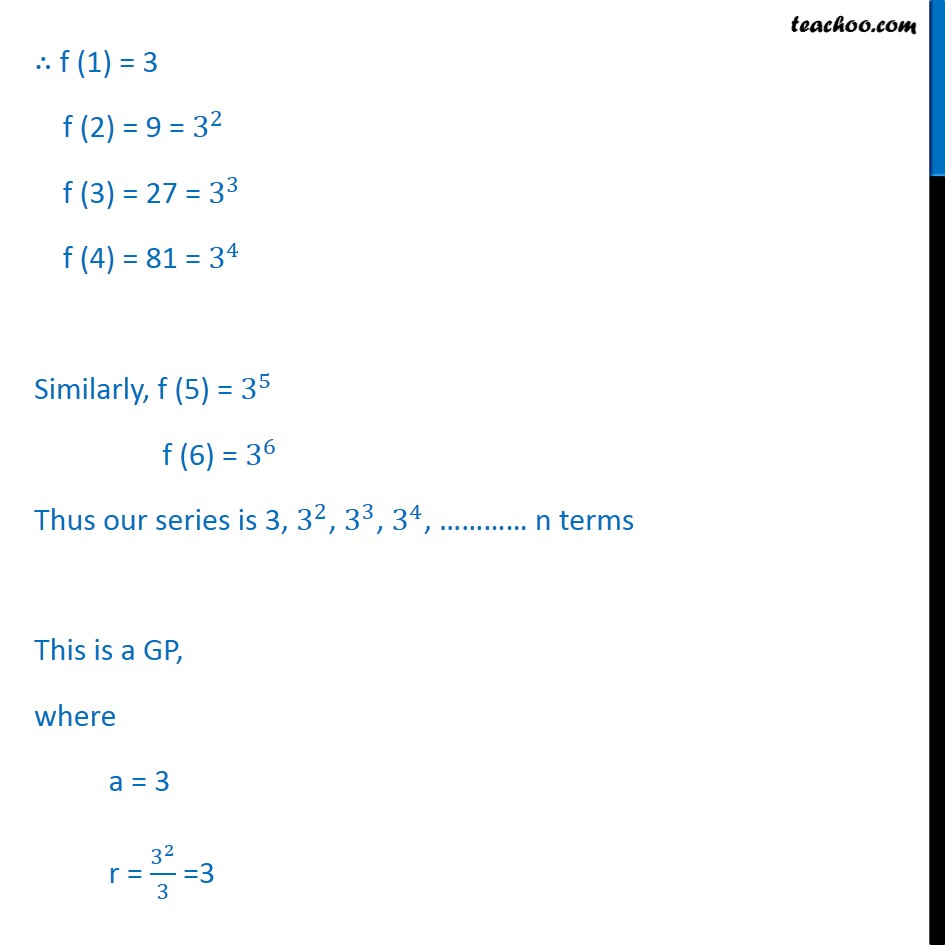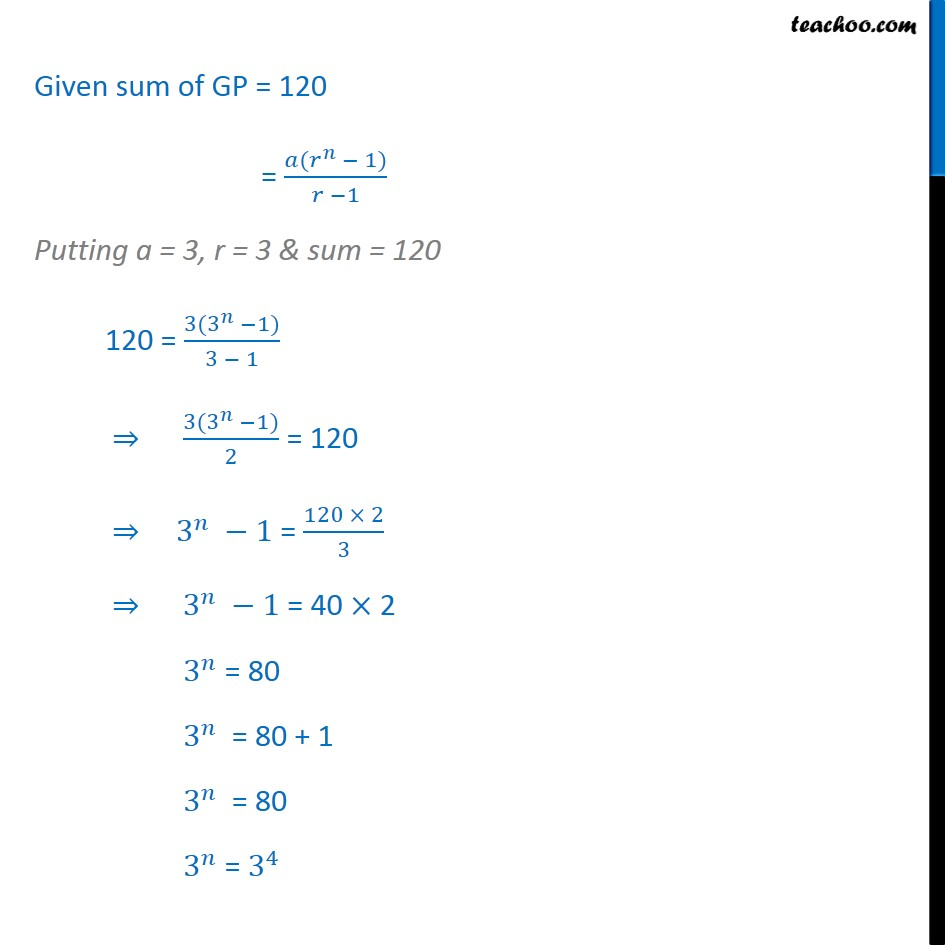Geometric Progression(GP): Formulae based

Chapter 8 Class 11 Sequences and Series
Concept wiseLearn in your speed, with individual attention - Teachoo Maths 1-on-1 Class

### Transcript

Misc 1 If f is a function satisfying f (x + y) = f(x) f(y) for all x, y N such that f(1) = 3 and , find the value of n. Given that : f (x + y) = f(x) f(y) x, y N and f(1) = 3 f (1) = 3 f (2) = 9 = 3 2 f (3) = 27 = 3 3 f (4) = 81 = 3 4 Similarly, f (5) = 3 5 f (6) = 3 6 Thus our series is 3, 3 2 , 3 3 , 3 4 , n terms This is a GP, where a = 3 r = 3 2 3 =3 Given sum of GP = 120 = ( 1) 1 Putting a = 3, r = 3 & sum = 120 120 = 3( 3 1) 3 1 3( 3 1) 2 = 120 3 1 = 120 2 3 3 1 = 40 2 3 = 80 3 = 80 + 1 3 = 80 3 = 3 4 n = 4 n = 4